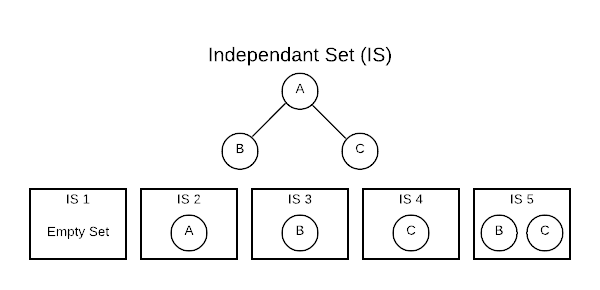Cut Property: If e is the cheapest edge crossing some cut (A, B), then e belongs to the MST

Clustering (aka unsupervised learning):

• Given $n$ points in space (web pages, images, genome fragments, database points, etc…) classify into “coherent groups”
• Assumptions
• as input, given a similarity measure - a distance $d(p,q)$ between point pair
• similarity measure is symmetric $d(p,q) = d(q,p)$
• Examples Euclidean distance, genome similarity, etc…
• Items in a cluster have a small distance

## Weighted Independent Set (WIS)

Conceptually, an Independent Set (IS) is comparable to the inverse of a graph. It’s a set of vertices that do not contain both endpoints of any edge. Consider a connected graph with only three vertices as shown below. There are five independent sets.The [] (empty set) is always considered an IS because it technically fits the definition. The sets [A], [B], and [C] are also ISs because no edge contains only one vertex. The set [B, C] also qualifies because there is no edges connecting B and C.

At first glance, this concept seems a bit academic; however, ISs are useful in practice. Finding an IS is a way of asking, which vertices do not have a relationship. For instance, if vertices are people and edges are familial relationship, an IS would define people who are not related.